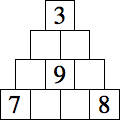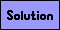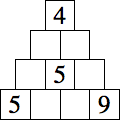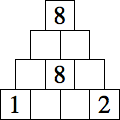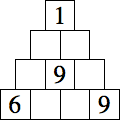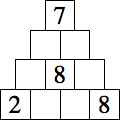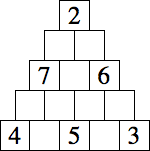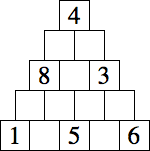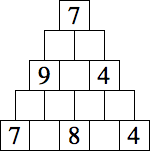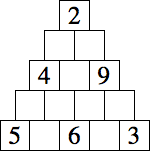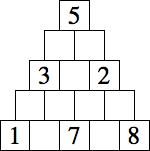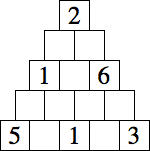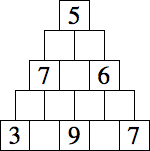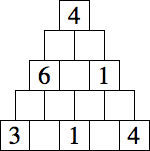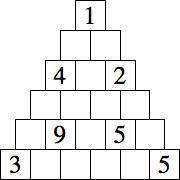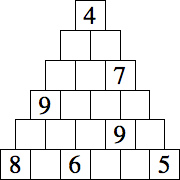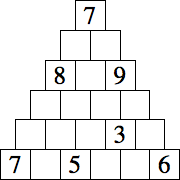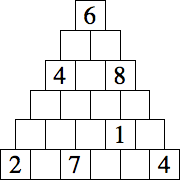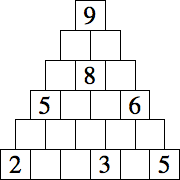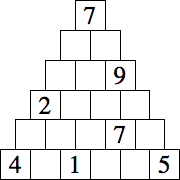Aztec Math Puzzles

Insert a digit 1-9 in each vacant square so that the number in each square is the sum, difference, product, or quotient of the numbers in the two squares immediately below it. The digits in each row will be different. An example is shown below.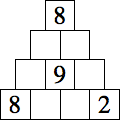→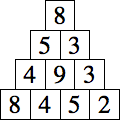Each puzzle below has a unique solution.